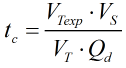# C131 – Sampler Control Interval Check

## Description

This equation calculates the time interval between samples.

KIMS calculation reference K161

Kelton calculation reference C131

## Calculation

All calculations are performed in SI units unless otherwise stated

The time interval between samples is calculated by:where:

 VTexp = Total export volume for the period VT = Total sample volume required VS = Volume of sample increment Qd = Displayed flowrate

Back